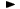ADVFN HomeHelpFinancialsDeeper AnalysisOPERATING RATIOS - Profitability RatiosGrowth from Plowback

# Growth from Plowback

Growth from Plowback ratio (or Sustainable Growth Rate), is the Plowback ratio multiplied by the Return on Equity (ROE). It measures roughly how rapidly the shareholders' investment is growing on an annual basis as a result of plowback. The formula is:

= Plowback Ratio * ROE
or
= [((EPS-basic - total annual dividend) / EPS-basic) * (attributable profit / average total ordinary capital & reserves)] * 100

Note: If the company had losses during the period under review, the ratio is not defined.